Capacitance of two parallel plates

The most common capacitor consists of two parallel plates. The capacitance of a parallel plate capacitor depends on the area of the plates A and their separation d. According to Gauss's law, the electric field between the two plates is: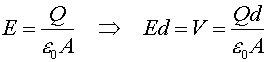Since the capacitance is defined by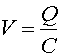one can see that capacitance is: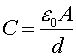Thus you get the most capacitance when the plates are large and close together. A large capacitance means that the capacitor stores a large amount of charge.

If a dielectric material is inserted between the plates, the microscopic dipole moments of the material will shield the charges on the plates and alter the relation. Materials have a permeability e which is given by the relative permeability k, e=ke0. The capacitance is thus given by: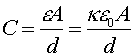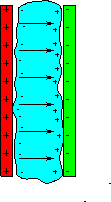All materials have a relative permeability, k, greater than unity, so the capacitance can be increased by inserting a dielectric. Sometimes, k is referred to as the dielectric constant of the material. The electric field causes some fraction of the dipoles in the material to orient themselves along the E-field as opposed to the usual random orientation. This, effectively, appears as if negative charge is lined up against the positive plate, and positve charge against the negative plate. In the figure to the right, the blue material is the dielectric.